# Transportation Problem | Set 3 (Least Cost Cell Method)

The North-West Corner method has been discussed in the previous article. In this article, the Least Cost Cell method will be discussed.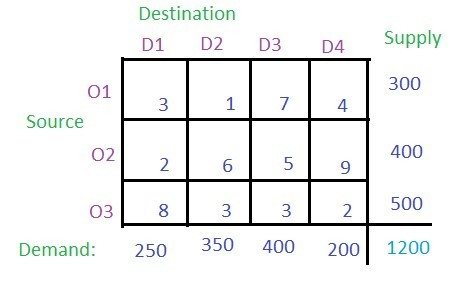Solution: According to the Least Cost Cell method, the least cost among all the cells in the table has to be found which is 1 (i.e. cell (O1, D2)).
Now check the supply from the row O1 and demand for column D2 and allocate the smaller value to the cell. The smaller value is 300 so allocate this to the cell. The supply from O1 is completed so cancel this row and the remaining demand for the column D2 is 350 – 300 = 50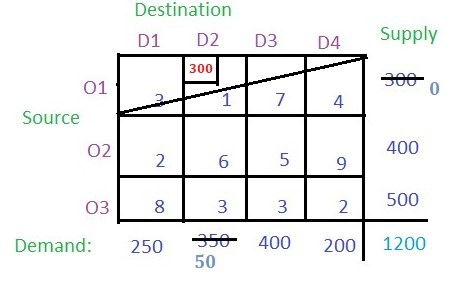Now find the cell with the least cost among the remaining cells. There are two cells with the least cost i.e. (O2, D1) and (O3, D4) with cost 2. Lets select (O2, D1). Now find the demand and supply for the respective cell and allocate the minimum among them to the cell and cancel the row or column whose supply or demand becomes 0 after allocation.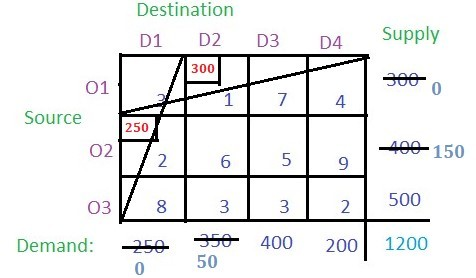Now the cell with the least cost is (O3, D4) with cost 2. Allocate this cell with 200 as the demand is smaller than the supply. So the column gets cancelled.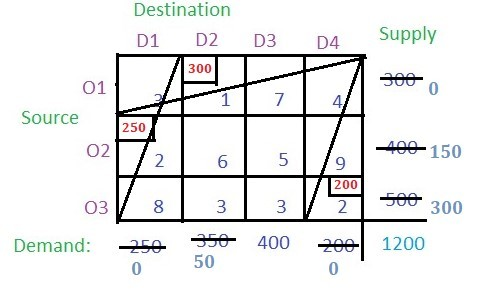There are two cells among the unallocated cells that have the least cost. Choose any at random say (O3, D2). Allocate this cell with a minimum among the supply from the respective row and the demand of the respective column. Cancel the row or column with zero value.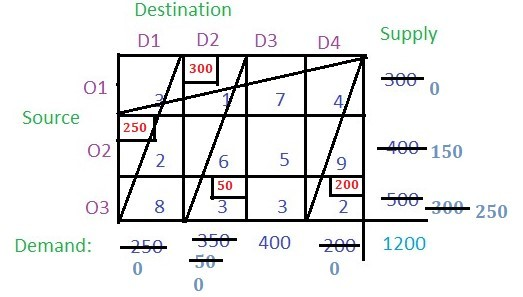Now the cell with the least cost is (O3, D3). Allocate the minimum of supply and demand and cancel the row or column with zero value.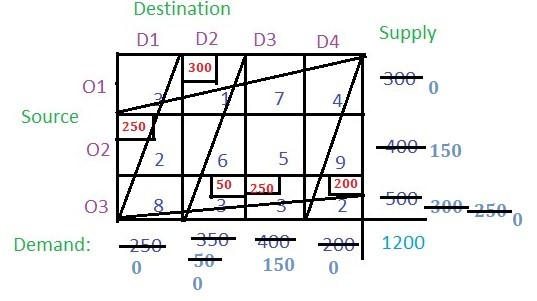The only remaining cell is (O2, D3) with cost 5 and its supply is 150 and demand is 150 i.e. demand and supply both are equal. Allocate it to this cell.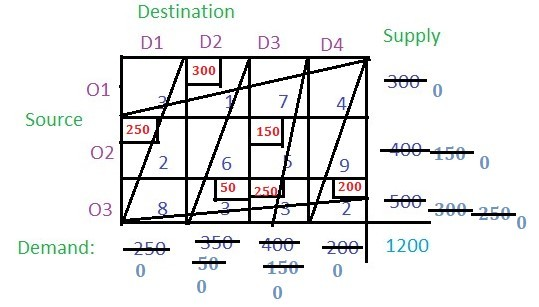Now just multiply the cost of the cell with their respective allocated values and add all of them to get the basic solution i.e. (300 * 1) + (250 * 2) + (150 * 5) + (50 * 3) + (250 * 3) + (200 * 2) = 2850

Feeling lost in the world of random DSA topics, wasting time without progress? It's time for a change! Join our DSA course, where we'll guide you on an exciting journey to master DSA efficiently and on schedule.
Ready to dive in? Explore our Free Demo Content and join our DSA course, trusted by over 100,000 geeks!